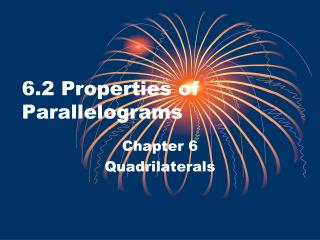DownloadDownload Presentation6.2 Properties of Parallelograms

# 6.2 Properties of Parallelograms

Download Presentation## 6.2 Properties of Parallelograms

- - - - - - - - - - - - - - - - - - - - - - - - - - - E N D - - - - - - - - - - - - - - - - - - - - - - - - - - -
##### Presentation Transcript

1. 6.2 Properties of Parallelograms Chapter 6 Quadrilaterals

2. 6.2 Properties of Parallelograms • Theorem 6-1: Opposite sides of a parallelogram are congruent

3. Consecutive and Opposite Angles • Consecutive Angles: Angles that share a side (Angles that are “next” to each other) • Opposite Angles: Angles that are across from each other in the parallelogram

4. 6.2 Properties of Parallelograms • Consecutive Angles are Supplementary because they are same-side interior angles

5. Using Consecutive Angles • Find m<S in RSTW ST and RW are parallel, so <S and <R are what kind of angles? S T 68° W R

6. 6.2 Properties of Parallelograms • Theorem 6-2: Opposite angles of a parallelogram are congruent

7. Using Algebra • Find the value of x in PQRS. Then find QR and PS. 3x - 15 R Q S P 2x + 3

8. Using Algebra • Find the value of y in EFGH. Then find m<E, m<F, m<G, m<H. E F 3y + 37 6y + 4 G H

9. 6.2 Properties of Parallelograms • Theorem 6-3: The diagonals of a parallelogram bisect each other

10. Using Algebra • Solve the system of equations to find the values of x and y. B C x + 1 2x 3y - 7 y D A

11. Solve:

12. Using Algebra • Solve the system of equations to find the values of x and y. Then find AE, EC, BE, and ED. B C x + 1 2x E 3y - 7 y D A

13. Using Algebra • Find the values of a and b. X Y a 2a - 8 V b + 10 b + 2 Z W

14. Theorem 6-4 • Theorem 6-4: If three (or more) parallel lines cut off congruent segments on one transversal, then they cut off congruent segments on every transversal.

15. Measurement • In the figure, DH ll CG ll BF ll AE, and AB = BC = CD = 2, and EF = 2.5. Find EH. H D 2 G C 2 F B 2 2.5 A E

16. Practice • Pg 297 1-21, 24-33